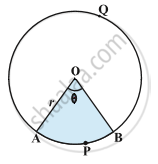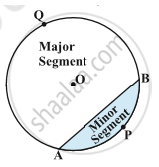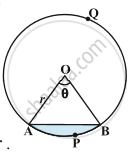# Areas of Sector and Segment of a Circle

#### description

• Area of the Sector and Circular Segment
• Length of an Arc

#### notes

1) Sector- The portion (or part) of the circular region enclosed by two radii and the corresponding arc is called a sector of the circle.Here, OAPB is the minor sector and OAQB is the major sector.Let OAPB be a sector of a circle with centre O and radius r. Let the degree measure of ∠AOB be θ.

Now, area of a circle= πr^2
We can consider this circular region to be a sector forming an angle of 360° at the centre O.
When angle at the centre is 360, area of the sector= πr^2
when angle at the centre is 1, area of the sector= (πr^2)/360
So, when angle at the centre is θ.
area of the sector= (πr^2)/360 xx θ
area of the sector= θ/360 xx πr^2

2) Segment- The potion (or part) of the circular region enclosed between a chord and the corresponding arc is called a segment of the circle.arc APB is the minor segment and arc AQB is the major segment.Here, in a circle with centre O and radius r, You can see that:
Area of the segment APB = Area of the sector OAPB- Area of ΔOAB
"Area"   "of"   "the"   "segment"   "APB" =θ/360 xx πr^2- "Area"   "of"   "ΔOAB"

If you would like to contribute notes or other learning material, please submit them using the button below.

### Shaalaa.com

Area Circles part 5 (Major Minor Sector and Segments) [00:10:18]
S
0%